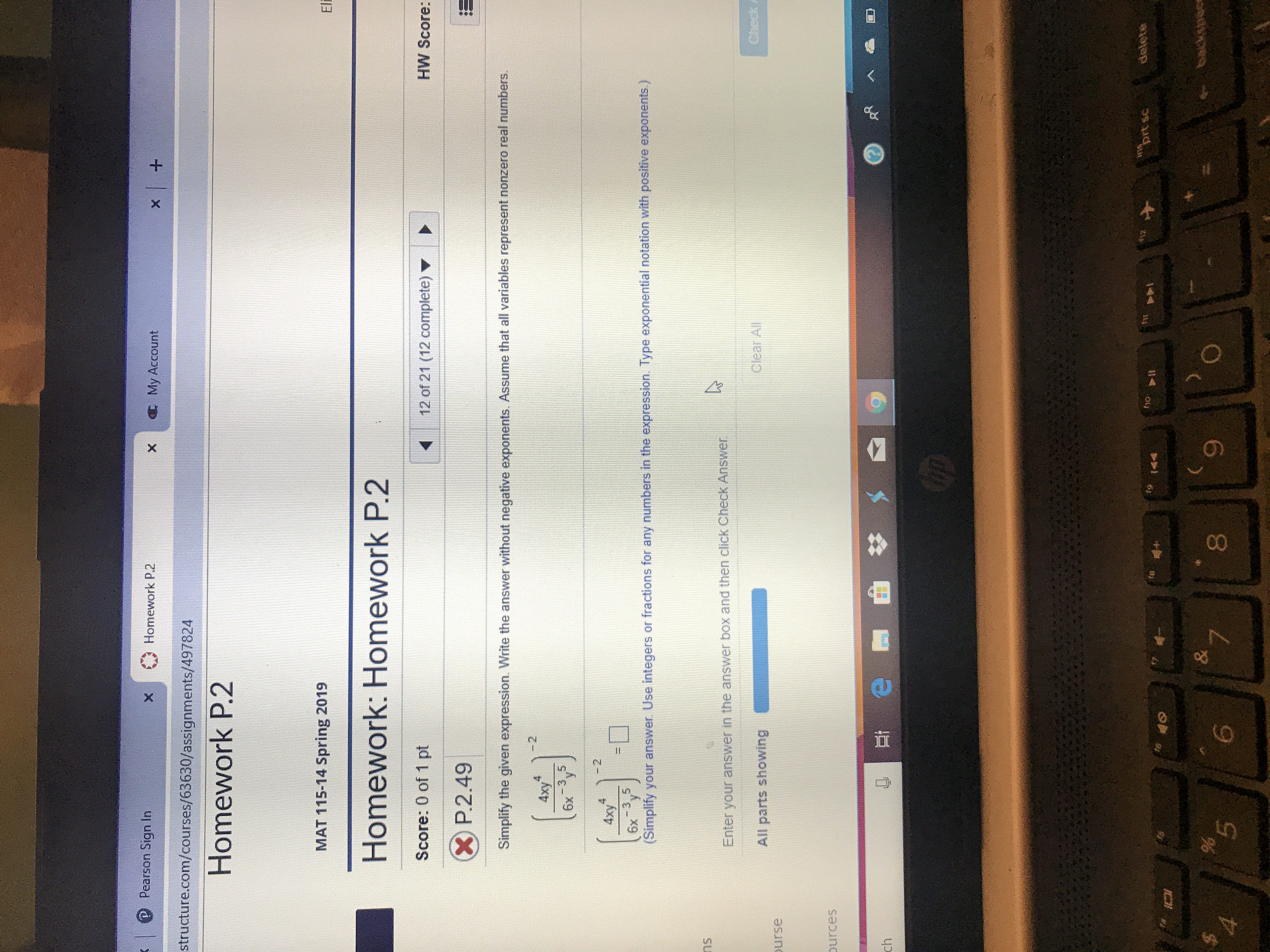# Pearson Sign Inx Homework P.2X My Accountstructure.com/courses/63630/assignments/497824Homework P.2MAT 115-14 Spring 2019Homework: Homework P.2Score: 0 of 1 pt12 of 21 (12 complete) ▼HW Score:P.2.49Simplify the given expression. Write the answer without negative exponents. Assume that all variables represent nonzero real numbers26x -3,52xy6x 3,5(Simplify your answer. Use integers or fractions for any numbers in the expression. Type exponential notation with positive exponents)nsEnter your answer in the answer box and then click Check AnswenAll parts showingClear Allurseurces85

Question

How do I answer this problem?help_outlineImage TranscriptionclosePearson Sign In x Homework P.2 X My Account structure.com/courses/63630/assignments/497824 Homework P.2 MAT 115-14 Spring 2019 Homework: Homework P.2 Score: 0 of 1 pt 12 of 21 (12 complete) ▼ HW Score: P.2.49 Simplify the given expression. Write the answer without negative exponents. Assume that all variables represent nonzero real numbers 2 6x -3,5 2 xy 6x 3,5 (Simplify your answer. Use integers or fractions for any numbers in the expression. Type exponential notation with positive exponents) ns Enter your answer in the answer box and then click Check Answen All parts showing Clear All urse urces 8 5 fullscreen
check_circleExpert Solution
Step 1

Given,

Step 2
Step 3

### Want to see the full answer?

See Solution

#### Want to see this answer and more?

Solutions are written by subject experts who are available 24/7. Questions are typically answered within 1 hour*

See Solution
*Response times may vary by subject and question
Tagged in

### Equations and In-equations